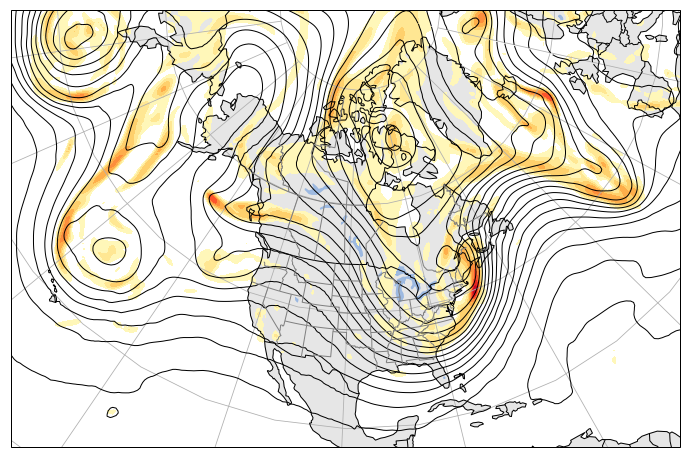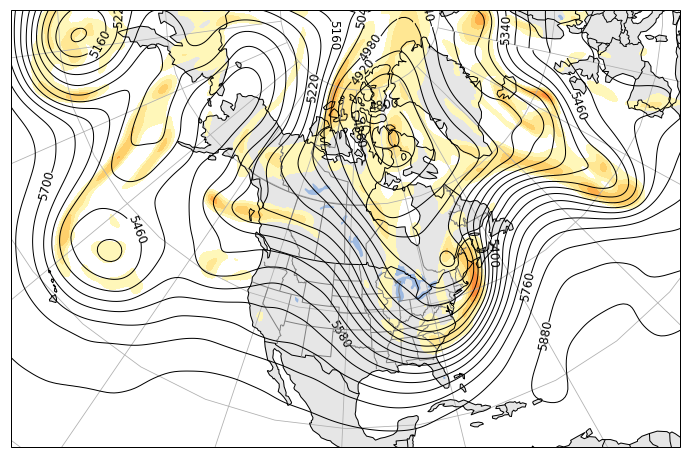# Importing and mapping reanalysis data with xarray and cartopy¶

### Christopher McCray - www.meteo.mcgill.ca/~cmccray¶

In this tutorial, we'll be using xarray to import NetCDF data, matplotlib to plot it, and cartopy to map it. I'll go over the basic usage of each package, with a few more functions from elsewhere thrown in at times.

First, we import the necessary packages (below). The plt.rcParams['figure.figsize'] just sets the size of the inline figures in this notebook to make them larger and easier to read.

In :
import xarray as xr
import cartopy.crs as ccrs
import cartopy.feature as cfeature
import matplotlib.pyplot as plt
import numpy as np
plt.rcParams['figure.figsize'] = (12, 12)


Let's load our data file. In this case, we have a NetCDF of Climate Forecast System Reanalysis (CFSR) pressure level data from 12 UTC 14 March 1993 on a 0.5° x 0.5° lat-lon grid (downloaded/subset from NCDC). Xarray provides a convenient interface for importing and analyzing NetCDF files.

Run the cell below to load the data and print out some basic metadata!

In :
dataFile = 'pgbh00.gdas.1993031412.nc'
#Open the dataset and print out metadeta
ds = xr.open_dataset(dataFile)
print ds

<xarray.Dataset>
Dimensions:                  (lat: 201, lon: 720, pressure: 37, time: 1)
Coordinates:
* time                     (time) datetime64[ns] 1993-03-14T12:00:00
* lat                      (lat) float64 90.0 89.5 89.0 88.5 88.0 87.5 ...
* lon                      (lon) float64 0.0 0.5 1.0 1.5 2.0 2.5 3.0 3.5 ...
* pressure                 (pressure) float64 1e+05 9.75e+04 9.5e+04 ...
Data variables:
Pressure_reduced_to_MSL  (time, lat, lon) float32 101394.0 101394.0 ...
Absolute_vorticity       (time, pressure, lat, lon) float32 0.0001215 ...
Geopotential_height      (time, pressure, lat, lon) float32 97.2256 ...
Temperature              (time, pressure, lat, lon) float32 239.3 239.3 ...
U-component_of_wind      (time, pressure, lat, lon) float32 3.19 3.14 ...
V-component_of_wind      (time, pressure, lat, lon) float32 -6.26 -6.29 ...
Attributes:
Conventions: CF-1.0
Originating_center: US National Weather Service (NCEP) subcenter = 0
Generating_Process_or_Model: Forecast
Product_Status: Operational products
Product_Type: Forecast products
cdm_data_type: GRID
creator_name: US National Weather Service (NCEP) subcenter = 0
file_format: GRIB-2
location: /modeldata/cmd_pgbh/1993/199303/19930314/pgbh00.gdas.1993031412.grb2
history: Direct read of GRIB-2 into NetCDF-Java 4.0 API
_CoordinateModelRunDate: 1993-03-14T12:00:00Z
History: Translated to CF-1.0 Conventions by Netcdf-Java CDM (NetcdfCFWriter)
Original Dataset = /modeldata/cmd_pgbh/1993/199303/19930314/pgbh00.gdas.1993031412.grb2; Translation Date = Sun Feb 05 20:31:29 UTC 2017


We can see that the dataset has 4 coordinates (time, lat, lon, and pressure) and 6 variables. We notice that the pressure coordinate is in Pascals. We can very easily convert these to hPa by dividing by 100, which will apply to all of the involved data.

In :
ds['pressure'] = ds['pressure']/100


Let's first plot 500-hPa heights and absolute vorticity. We pull out some necessary fields, including our lat/lon coordinates for mapping. To index on coordinate values in xarray, use the .sel command. To index based on position (starting with 0), use isel. Since we only have one time value in this case, we select time=0.

In :
lat = ds['lat']
lon = ds['lon']
hght = ds['Geopotential_height'].sel(pressure=500).isel(time=0)
avor = ds['Absolute_vorticity'].sel(pressure=500).isel(time=0)


### Mapping with cartopy¶

Cartopy makes mapping fairly intuitive and easy! First, we define our coordinate reference system (ccrs) and choose a projection from those available. We then create a figure and pass the chosen projection information to it (in this case, a Lambert Conformal conic projection) to create what's known as a GeoAxes instance. This is where we'll map all of our data. We can use set_extent to zoom in on a certain lat/lon box.

We can then quickly add various features to the map, including a land background, lakes, country/state/provincial borders, etc.

Here, I've set this all up as a function plotMap() which, when run, will create and return a figure with a background map on which to plot without the need to copy/paste all of this code each time.

Note that I've added a zorder argument to some features. zorder tells matplotlib where to draw the feature relative to the other parts of the plot, with 0 being the bottommost layer and increasing values being closer to the top layer of the figure.

In :
def plotMap():
#Set the projection information
proj = ccrs.LambertConformal(central_longitude=-97.0,central_latitude=53, standard_parallels=)
#Create a figure with an axes object on which we will plot. Pass the projection to that axes.
fig, ax = plt.subplots(subplot_kw=dict(projection=proj))

#Zoom in
ax.set_extent([-45, -155, 10, 90])

ax.add_feature(cfeature.LAND, facecolor='0.9') #Grayscale colors can be set using 0 (black) to 1 (white)
ax.add_feature(cfeature.LAKES, alpha=0.9)  #Alpha sets transparency (0 is transparent, 1 is solid)

#We can use additional features from Natural Earth (http://www.naturalearthdata.com/features/)
states_provinces = cfeature.NaturalEarthFeature(
scale='50m', facecolor='none')

#Add lat/lon gridlines every 20° to the map
ax.gridlines(xlocs=np.arange(0,361,20), ylocs=np.arange(-80,90,20))

return fig, ax


Now, let's plot our data! We first call plotMap() to get our background map and figure. Then, we specify the interval for our height contours. We use numpy's function arange to select a range of contours from 4320 to 6000 m, every 60 m (6 dam, the standard for 500-hPa. Note that the height field can easily be converted from meters to decameters in the way we converted the pressure coordinate above). Running hght.min() and hght.max() will show us that min and max values for 500-hPa heights in the dataset fall well within the range we've chosen to plot.

A key thing to note is the transform argument at the end of the contour call. This is what tells matplotlib to transform our variable in lat/lon coordinates onto our map projection coordinates.

We then plot absolute vorticity in filled contours. Matplotlib has many colormaps from which to choose. Here, we use YlOrRd (yellow-orange-red).

In :
#Get a new background map figure
fig, ax = plotMap()

#Plot the 500-hPa height contours on the map, in black, with line width 1, and plot it above everything else.
hght_levels = np.arange(4320,6000,60)
ax.contour(lon, lat,hght, colors='k', levels=hght_levels, linewidths=1, zorder=3, transform = ccrs.PlateCarree())

#Now plot absolute vorticity as filled contours underneath height field, only values above 1.5e-4 s-1,
# and use the YlOrRd colormap.
avor_levels = np.linspace(15e-5,60e-5, 10)
avor_contour = ax.contourf(lon, lat, avor, levels = avor_levels,
cmap=plt.cm.YlOrRd, zorder=2, transform = ccrs.PlateCarree())

#Show the figure
fig

Out:Great! Since fields can be somewhat noisy (particularly vorticity), we can smooth out the map a bit by using a Gaussian filter on the data (like the GWFS function in GEMPAK). To do this, we can use SciPy's Guassian filter function.

Additionally, we can add labels to our contours with clabel. We can format the labels as integers (fmt='%1i') so that decimals don't appear.

In :
#Import scipy.ndimage to get the gaussian_filter function
import scipy.ndimage as ndimage

#Plot a new figure and map axes
fig, ax = plotMap()

#Smooth and re-plot the vorticity field
avor_smooth = ndimage.gaussian_filter(avor, sigma=1.5, order=0)
avor_contour = ax.contourf(lon, lat, avor_smooth, levels = avor_levels, zorder=2,
cmap=plt.cm.YlOrRd, transform = ccrs.PlateCarree())

#Smooth and re-plot the height field
hght_smooth = ndimage.gaussian_filter(hght, sigma=3, order=0)
hght_contour = ax.contour(lon, lat, hght_smooth, levels=hght_levels, linewidths=1, colors='k',
zorder=11,transform = ccrs.PlateCarree())

#Plot contour labels for the heights, leaving a break in the contours for the text (inline=True)
plt.clabel(hght_contour,  hght_levels, inline=True, fmt='%1i', fontsize=12)

fig

Out:Let's add a colorbar for avor. Since the actual values have many leading zeros, we want to make the colorbar tick labels a bit more visually appealing. There are many ways to do this. One quick way is to simply multiply the tick label values, which are really our avor_levels values from above, by $10^5$

Let's also set a title. To get mathematical notations, we can use LaTeX-esque syntax between two $. Use the newline character \n to add a second line. In : #Create a colorbar and shrink it down a bit. cb = plt.colorbar(avor_contour, shrink=0.5) #Change the tick labels cb.set_ticklabels(avor_levels*10**5) #Set the title ax.set_title('500-hPa Heights, Absolute Vorticity$10^{-5} s^{-1}\$\n 1993-03-14 12UTC', fontsize=14)

fig

Out: